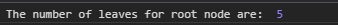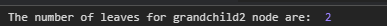# D3.js node.count() Function

• Last Updated : 23 Sep, 2020

The node.count() function of D3.js library is used to count the number of leaves under a particular node and append it as a value property to the object. If the node given is itself a leaf node then the count is one.

Syntax:

`node.count();`

Parameters: This function does not take any parameter.

Return Value: This function returns an object.

Below given are a few examples of the function given above.

Example 1: The following example demonstrates counting leaves for the root node.

## HTML

 ```<``html` `lang``=``"en"``>`` ` `<``head``>``    ``<``meta` `charset``=``"UTF-8"` `/>``    ``<``meta` `name``=``"viewport"` `path1tent``=``    ``"width=device-width, initial-scale = 1.0"` `/>`` ` `    ``<``script` `src``=``"https://d3js.org/d3.v4.min.js"``>``    `````` ` `<``body``>``    ``<``script``>``        ``// Constructing a tree``        ``var tree = {``            ``name: "rootNode",``            ``children: [``                ``{``                    ``name: "child1"``                ``},``                ``{``                    ``name: "child2",``                    ``children: [``                        ``{``                            ``name: "grandchild1",``                            ``children: [``                                ``{ name: "grand_granchild1_1" },``                                ``{ name: "grand_granchild1_2" }``                            ``]``                        ``},``                        ``{``                            ``name: "grandchild2",``                            ``children: [``                                ``{ name: "grand_granchild2_1" },``                                ``{ name: "grand_granchild2_2" }``                            ``]``                        ``},``                    ``]``                ``}``            ``]``        ``};`` ` `        ``var obj = d3.hierarchy(tree);`` ` `        ``// Using node.count() Function``        ``var count = obj.count();``        ``console.log("The number of leaves for root"``                ``+ " node are: ", count.value);``    `````` ` ``

Output:Example 2: The following code demonstrates counting number of leaves for any node.

## HTML

 ```<``html` `lang``=``"en"``>`` ` `<``head``>``    ``<``meta` `charset``=``"UTF-8"` `/>``    ``<``meta` `name``=``"viewport"` `path1tent``=``        ``"width=device-width, initial-scale = 1.0"` `/>``    ``<``script` `src``=``"https://d3js.org/d3.v4.min.js"``>``    `````` ` `<``body``>``    ``<``script``>``        ``// Constructing a tree``        ``var tree = {``            ``name: "rootNode", // Root node``            ``children: [``                ``{``                    ``name: "child1" // Child of root node``                ``},``                ``{``                    ``name: "child2", // Child of root node``                    ``children: [``                        ``{``                            ``// Child of child2``                            ``name: "grandchild1",``                            ``children: [``                                ``// Child of grandchild1``                                ``{ name: "grand_granchild1_1" },``                                ``// Child of grandchild1 ``                                ``{ name: "grand_granchild1_2" }``                            ``]``                        ``},``                        ``{``                            ``name: "grandchild2",``                            ``children: [``                                ``// Child of grandchild2``                                ``{ name: "grand_granchild2_1" },``                                ``// Child of grandchild2``                                ``{ name: "grand_granchild2_2" }``                            ``]``                        ``},``                    ``]``                ``}``            ``]``        ``};`` ` `        ``var obj = d3.hierarchy(tree);``        ``var grandchild2 = obj.children.children;`` ` `        ``// Using node.count() function``        ``var count = grandchild2.count();``        ``console.log("The number of leaves for "``            ``+ "grandchild2 are: ", count.value);``    `````` ` ``

Output:My Personal Notes arrow_drop_up